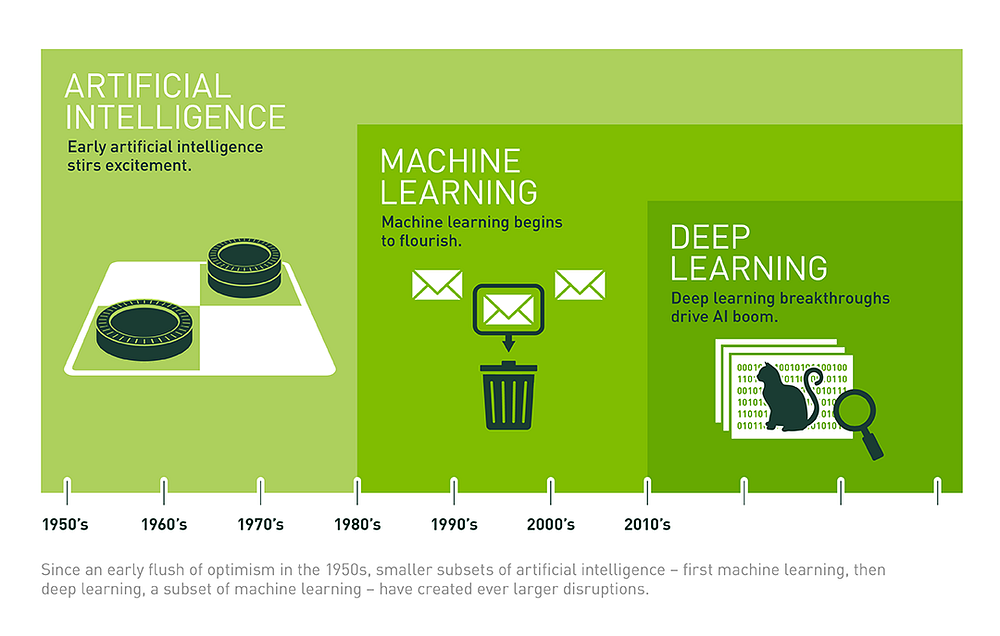# Machine Learning & Deep Learning Guide – Part 1

Source: Deep Learning on Medium# Supervised learning: Linear Regression:

1. Data definition: We will use Boston Housing Data. We want to predict the house prices based on some attributes such as per capita crime rate by town, the proportion of residential land zoned for lots over 25,000 sq.ft, average number of rooms per dwelling and others. I downloaded the file and renamed it to boston.csv and added the following line as a header of the file:
`# Load the diabetes datasetimport pandas as pdboston = pd.read_csv('data/boston.csv')y = boston['MEDV']X = boston.drop('MEDV',axis=1)#View the top 5 rowsboston.head())`

2. Train/Test split: As the dataset is very small, we will split it into 10% for testing and 90% for training

`# Split train and test setfrom sklearn.model_selection import train_test_splitX_train,X_test,y_train,y_test = train_test_split(X,y,test_size= 0.1, random_state=0)# View the shape (structure) of the dataprint(f"Training features shape: {X_train.shape}")print(f"Testing features shape: {X_test.shape}")print(f"Training label shape: {y_train.shape}")print(f"Testing label shape: {y_test.shape}")`

Result:
Training features shape: (455, 13)
Testing features shape: (51, 13)
Training label shape: (455,)
Testing label shape: (51,)

3. Preprocessing: We didn’t need to do any data preprocessing. But it will be applied in later examples.

4. Algorithm Selection: We will use LinearRegression

`#Linear Regressionfrom sklearn.linear_model import LinearRegressionfrom sklearn.metrics import mean_squared_error, r2_score,mean_absolute_errorlr = LinearRegression(normalize=True)`

5. Training: Simply we call the function fit and give it X_train and y_train as parameters.

`lr.fit(X_train, y_train) # Fit the model to the data`

6. Prediction: Simply we call predict

`y_pred_lr = lr.predict(X_test) # Predict labels`

7. Evaluate Model’s Performance:

`# The mean squared errorprint(f"Mean squared error: { mean_squared_error(y_test, y_pred_lr)}")# Explained variance score: 1 is perfect predictionprint(f"Variance score: {r2_score(y_test, y_pred_lr)}")# Mean Absolute Errorprint(f"Mean squared error: { mean_absolute_error(y_test, y_pred_lr)}")`

Result:
Mean squared error: 41.72457625585755
Variance score: 0.5149662051867079
Mean squared error: 3.9357920841192797

8. Fine Tuning: At this stage we will not do any fine tuning. To keep things smooth and simple.